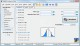ESBPDF Analysis - Probability Software 2.4.1 Probability Analysis Software for Windows that is easy to use. Shareware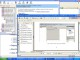WebCab Probability and Stat for Delphi 3.6 Statistics, Discrete Prob, Distributions, Hypo. testing, Correlation,Regression DemoWebCab Probability and Stat (J2EE Ed.) 3.6 Statistics, Discrete Prob, Distributions, Hypo. testing, Correlation,Regression DemoWebCab Probability and Stat for .NET 3.3 Statistics, Discrete Prob, Distributions, Hypo. testing, Correlation,Regression DemoWebCab Probability and Stat (J2SE Ed.) 3.3 Statistics, Discrete Prob, Distributions, Hypo. testing, Correlation,Regression Demo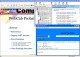WebCab Probability and Stat for .NET 3.6 Statistics, Discrete Prob, Distributions, Hypo. testing, Correlation,Regression Demo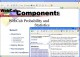WebCab Probability and Stat (J2SE Ed.) 3.6 Statistics, Discrete Prob, Distributions, Hypo. testing, Correlation,Regression Demo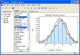EasyFit 5.0 Analyze your probability data and apply the results to make business decisions. CommercialDomino Probability 1.0 Students are shown a set of dominos and asked the probability of a certain outcome, such as picking a domino whose two numbers sum to eight. Freeware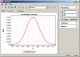StatAssist 2.0 View distribution graphs, calculate stat. moments, quantiles, and probabilities. Shareware